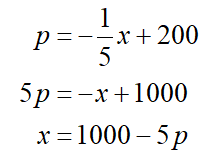# The price p, in dollars, of a certain commodity and the quantity x sold obey the demandequation p = −1/5x + 200 where 0 ≤ x ≤ 1000Suppose that the cost C, in dollars, of producing x units is C =√x/10+ 400Assuming that all items produced are sold, find the cost C as a function of the price p.(ii)The profit function, in dollars, for a product is given byΠ(x) = −x^3 + 76x^2 − 380x − 2800,where x is the number of units produced and sold. If break-even occurs when 10 units areproduced and sold,(a) Find a quadratic factor of Π(x).(b) Find a number of units other than 10 that gives break-even for the product.

Question
31 views

The price p, in dollars, of a certain commodity and the quantity x sold obey the demand
equation p = −1/5x + 200 where 0 ≤ x ≤ 1000

Suppose that the cost C, in dollars, of producing x units is C =√x/10+ 400
Assuming that all items produced are sold, find the cost C as a function of the price p.

(ii)
The profit function, in dollars, for a product is given by
Π(x) = −x^3 + 76x^2 − 380x − 2800,
where x is the number of units produced and sold. If break-even occurs when 10 units are
produced and sold,
(a) Find a quadratic factor of Π(x).
(b) Find a number of units other than 10 that gives break-even for the product.

check_circle

Step 1

Hi there! Thank you for the question, but as per our guidelines we can solve only one question at a time. We’ll answer the first question for you. Please submit a new question specifying the one you would like to get answered.

Step 2

Consider the demand equation

p = −1/5x + 200 where 0 ≤ x ≤ 1000

Step 3

Solve for x as a function of p...

### Want to see the full answer?

See Solution

#### Want to see this answer and more?

Solutions are written by subject experts who are available 24/7. Questions are typically answered within 1 hour.*

See Solution
*Response times may vary by subject and question.
Tagged in